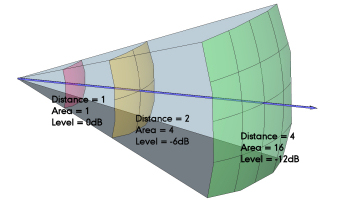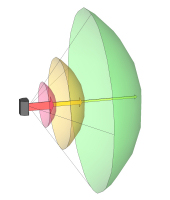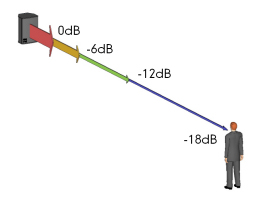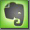Inverse Square Law

Any point source which spreads its influence equally in all directions without a limit to its range will obey the inverse square law. This comes from strictly geometrical considerations. The intensity of the influence at any given radius r is the source strength divided by the area of the sphere. Being strictly geometric in its origin, the inverse square law applies to diverse phenomena. Point sources of gravitational force, electric field, light, sound or radiation obey the inverse square law.The inverse-square law is a principle that expresses the way radiant energy propagates through space. The rule states that the power intensity per unit area from a point source, if the rays strike the surface at a right angle, varies inversely according to the square of the distance from the source. (Source: Whatis?com)

The Inverse-Square Law in Sound Propagation

The sound pressure level diminishes inversely with the square of the distance from the source (as all other forces).
If the distances from the sound source are d1 and d2,  then the difference Dd in dB at these points is: Dd = 10 log (d2/d1)2 = 20 log (d2/d1)(feet or meters)
 Distance 1 from the sound source Distance 2 from the sound source Result: Level drop at position 2

Speed of Sound

C = (331,4 + 0,6n) [m/s]

C = Speed of Sound, n = Temperatur